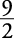## Rational Zero Theorem

If a polynomial function, written in descending order of the exponents, has integer coefficients, then any rational zero must be of the form ± p/ q, where p is a factor of the constant term and q is a factor of the leading coefficient.

##### Example 1

Find all the rational zeros of

f ( x) = 2 x 3 + 3 x 2 – 8 x + 3

According to the rational zero theorem, any rational zero must have a factor of 3 in the numerator and a factor of 2 in the denominator.The possibilities of p/ q, in simplest form, areThese values can be tested by using direct substitution or by using synthetic division and finding the remainder. Synthetic division is the better method because if a zero is found, the polynomial can be written in factored form and, if possible, can be factored further, using more traditional methods.

##### Example 2

Find rational zeros of f(x) = 2 x 3 + 3 x 2 – 8 x + 3 by using synthetic division.

Each line in the table that follows represents the “quotient line” of the synthetic division.

p/q

2

3

–8

3

1

2

5

–3

0

1 is a zero.

–1

2

1

–9

122

4

–6

0is a zero.2

2

–93

2

9

19

60

–3

2

–3

1

0

–3 is a zero.2

6

12

0

–8

15

The zeros of f ( x) = 2 x 3 + 3 x 2 – 8 x + 3 are 1,, and –3. This means

f (1) = 0,, and  f (–3) = 0

The zeros could have been found without doing so much synthetic division. From the first line of the chart, 1 is seen to be a zero. This allows f ( x) to be written in factored form using the synthetic division result.

f ( x) = 2 x 3 + 3 x 2 – 8 x + 3 = ( x – 1)(2 x 2 + 5 x – 3)

But 2 x 2 + 5 x – 3 can be further factored into (2 x – 1)( x + 3) using the more traditional methods of factoring.

2 x 2 + 5 x – 3 = ( x – 1)(2 x – 1)( x + 3)

From this completely factored form, the zeros are quickly recognized. Zeros will occur whenBack to Top
A18ACD436D5A3997E3DA2573E3FD792A